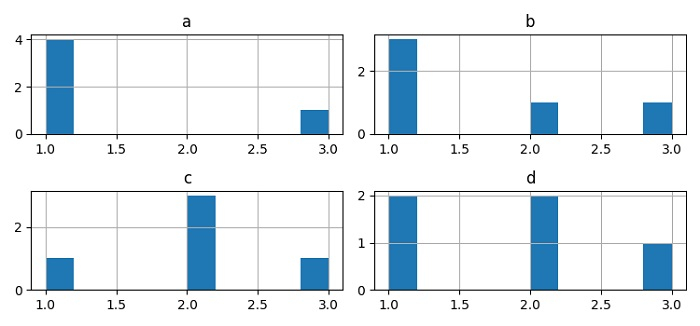# Plotting histograms against classes in Pandas / Matplotlib

MatplotlibPythonData Visualization

#### Python Data Science basics with Numpy, Pandas and Matplotlib

Most Popular

63 Lectures 6 hours

#### Data Visualization using MatPlotLib & Seaborn

11 Lectures 4 hours

#### MatPlotLib with Python

9 Lectures 2.5 hours

To plot histograms against classes in Pandas/Matplotlib, we can take the following steps −

• Set the figure size and adjust the padding between and around the subplots.

• Make a potentially hetrogeneous tabular data using Pandas dataframe.

• Make a histogram from the DataFrame values.

• To display the figure, use show() method.

## Example

from matplotlib import pyplot as plt
import pandas as pd

plt.rcParams["figure.figsize"] = [7.50, 3.50]
plt.rcParams["figure.autolayout"] = True
df = pd.DataFrame({'a': [1, 1, 1, 1, 3],'b': [1, 1, 2, 1, 3],'c': [2, 2, 2, 1, 3],
'd': [2, 1, 2, 1, 3],})

df.hist()
plt.show()

## Output